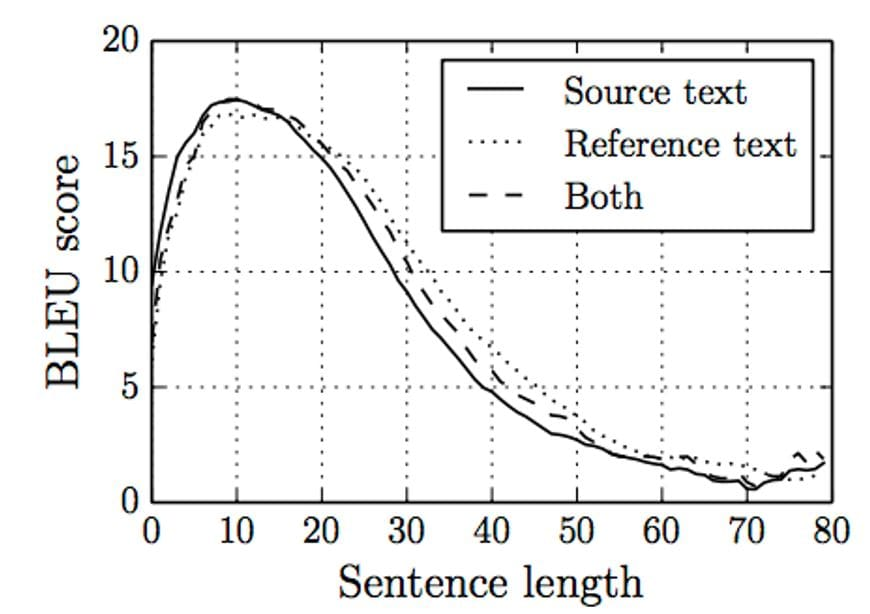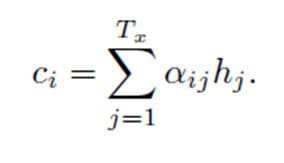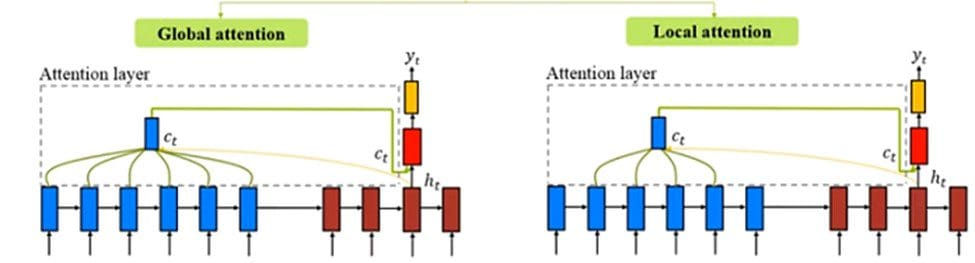## Attention mechanism in Deep Learning, Explained

[ad_1]

Attention is one of the most prominent ideas in the Deep Learning community. Even though this mechanism is now used in various problems like image captioning and others, it was originally designed in the context of Neural Machine Translation using Seq2Seq Models.

### Seq2Seq model

The seq2seq model is normally composed of an encoder-decoder architecture, where the encoder processes the input sequence and encodes/compresses the information into a context vector (or “thought vector”) of fixed length. This representation is anticipated to be a good summary of the complete input sequence. The decoder is then initialized with this context vector, using which it starts producing the transformed or translated output.

### Disadvantage of Seq2Seq model

A critical disadvantage of this fixed-length context vector design is the inability of the system to retain longer sequences. Often it has forgotten the earlier elements of the input sequence once it has processed the complete sequence. The attention mechanism was created to resolve this problem of long dependencies.Image source.

BLEU (Bilingual Evaluation Understudy) is a score for comparing a candidate translation of text to one or more reference translations. The above graph shows that the encoder-decoder unit fails to memorize the whole long sentence. Hence, what’s reflected from the graph above is that the encoder-decoder unit works well for shorter sentences (high bleu score).

### The basic idea behind Attention

Attention was presented by Dzmitry Bahdanau, et al. in their 2014 paper “Neural Machine Translation by Jointly Learning to Align and Translate,” which reads as a natural extension of their previous work on the Encoder-Decoder model. This very paper laid the foundation of the famous paper Attention is All You Need by Vaswani et al., on transformers that revolutionized the deep learning arena with the concept of parallel processing of words instead of processing them sequentially.

So coming back, the core idea is each time the model predicts an output word, it only uses parts of the input where the most relevant information is concentrated instead of the entire sequence. In simpler words, it only pays attention to some input words.

Attention is an interface connecting the encoder and decoder that provides the decoder with information from every encoder hidden state. With this framework, the model is able to selectively focus on valuable parts of the input sequence and hence, learn the association between them. This helps the model to cope efficiently with long input sentences.

### Intuition

The below figure demonstrates an Encoder-Decoder architecture with an attention layer.Source: Feed the context vector to decoder.

The idea is to keep the decoder as it is, and we just replace sequential RNN/LSTM with bidirectional RNN/LSTM in the encoder.

Here, we give attention to some words by considering a window size Tx (say four words x1, x2, x3, and x4). Using these four words, we’ll create a context vector c1, which is given as input to the decoder. Similarly, we’ll create a context vector c2 using these four words. Also, we have α1, α2, and α3 as weights, and the sum of all weights within one window is equal to 1.

Similarly, we create context vectors from different sets of words with different α values.

The attention model computes a set of attention weights denoted by α(t,1), α(t,2),..,α(t,t) because not all the inputs would be used in generating the corresponding output. The context vector ci for the output word yi is generated using the weighted sum of the annotations:The attention weights are calculated by normalizing the output score of a feed-forward neural network described by the function that captures the alignment between input at j and output at i.### Implementation

Let’s take an example where a translator reads the English(input language) sentence while writing down the keywords from the start till the end, after which it starts translating to Portuguese (the output language). While translating each English word, it makes use of the keywords it has understood.

Attention places different focus on different words by assigning each word with a score. Then, using the softmax scores, we aggregate the encoder hidden states using a weighted sum of the encoder hidden states to get the context vector.

The implementations of an attention layer can be broken down into 4 steps.

Step 0: Prepare hidden states.

First, prepare all the available encoder hidden states (green) and the first decoder hidden state (red). In our example, we have 4 encoder hidden states and the current decoder hidden state. (Note: the last consolidated encoder hidden state is fed as input to the first time step of the decoder. The output of this first time step of the decoder is called the first decoder hidden state.)

Step 1: Obtain a score for every encoder hidden state.

A score (scalar) is obtained by a score function (also known as alignment score function or alignment model). In this example, the score function is a dot product between the decoder and encoder hidden states.

Step 2: Run all the scores through a softmax layer.

We put the scores to a softmax layer so that the softmax scores (scalar) add up to 1. These softmax scores represent the attention distribution.

Step 3: Multiply each encoder hidden state by its softmax score.

By multiplying each encoder hidden state with its softmax score (scalar), we obtain the alignment vector or the annotation vector. This is exactly the mechanism where alignment takes place.

Step 4: Sum the alignment vectors.

The alignment vectors are summed up to produce the context vector. A context vector is an aggregated information of the alignment vectors from the previous step.

Step 5: Feed the context vector into the decoder.

### Types of attention

Depending on how many source states contribute while deriving the attention vector (α), there can be three types of attention mechanisms:Image from EazyLearn.

• Global Attention: When attention is placed on all source states. In global attention, we require as many weights as the source sentence length.
• Local Attention: When attention is placed on a few source states.
• Hard Attention: When attention is placed on only one source state.

You can check out my Kaggle notebook or GitHub repo to implement NMT with the attention mechanism using TensorFlow.

Related:

Read More …

[ad_2]